# Bash Script – Working of Bash Variables

• Last Updated : 06 May, 2022

A Bash script is a plain text file. This file contains different commands for step-by-step execution. These commands can be written directly into the command line but from a reusability perceptive it is useful to store all of the inter-related commands for a specific task in a single file. We can use that file for executing the set of commands one or more times as per our requirements. In programming, a variable is a value that can change, depending on conditions or on information passed to the program. A variable in bash can contain a number, a character, or a string of characters. Here in this article, we are going to discuss the working of variables within Bash Scripting.

Rules for defining variables in Bash Scripts are as follows –

• Variable names can contain uppercase, lowercase letters, numbers, underscores, and digits.
• It is a good practice to use uppercase letters for variable names within Bash scripts.
• Space is not allowed.
• Pre-defined keywords cannot be used. Like if, else, etc.

The simplest example of the use of variables in Bash scripting can be given as –

Example Script:

```NAME="Satyajit Ghosh"
echo "His name is \$NAME"```

Output:

`His name is Satyajit Ghosh`

The above example shows a variable ‘name‘. Then it is used with an echo command to display its value. So, the basic syntax for writing variables within a Bash Script will be –

Syntax of Writing Variables:

```VariableName=value
echo \$VariableName #for accessing the value```

If we want to change the value of a variable then we can do that. The basic syntax for that will be –

```VariableName=value
VariableName=newValue
echo \$VariableName #for accessing the value```

Below is an example of the same –

Example Script:

```AGE=10 #assigning a value
AGE=20 #changing the value
echo \$AGE #display the value```

Output:

`20`

Below is the terminal shell depiction after executing the script –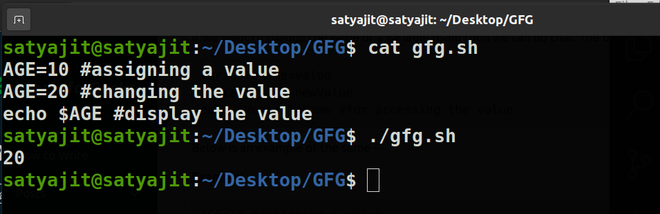## Variable Scope

Scope in a program or script is a region where the variables have their existence. If a variable, is declared inside a function then it is generally a local variable and if it is declared outside then it is a global variable. In the case of a bash script, this concept is a little bit different, here any variable whether it is written inside a function or outside a function by default is a global variable. If we want to make a local variable then we need to use the keyword “local”.

An example of the same is given below –

Example Script:

```#!/bin/bash

var1="Apple" # global variable
myfun(){
local var2="Banana" #local variable
var3="Cherry" #global variable
echo "The name of first fruit is \$var1"
echo "The name of second fruit is \$var2"
}
myfun

echo "The name of first fruit is \$var1"

# trying to access local variable
echo "The name of second fruit is \$var2"
echo "The name of third fruit is \$var3"```

Output of Variable Scope:

```The name of first fruit is Apple
The name of second fruit is Banana
The name of first fruit is Apple
The name of second fruit is
The name of third fruit is Cherry```

Here in this above example, var2 is a local variable, so when we are accessing it from the function it is doing fine but when we are trying to access it outside the function, it is giving us an empty result in the output.

On the other hand, unlike programming languages, even though var3 is defined inside a function still it is acting as a global variable and it can be accessed outside the function. Below is the terminal shell depiction after executing the script –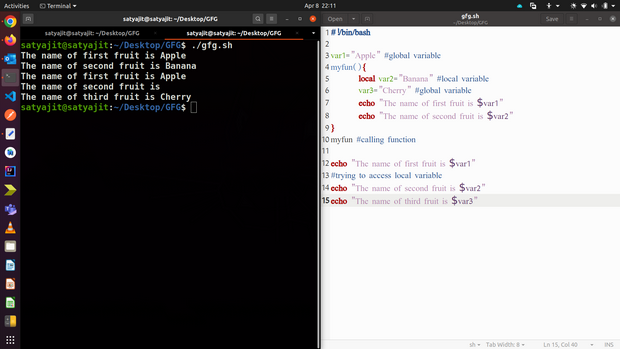## Operations on Variables

We can perform both numerical and string operations on variables on Bash scripting. An example of the same is given below –

Example Script:

```NUM1=10 #variable 1
NUM2=5 #variable 2

# Numerical Operations over the variables
SUM=\$(( \$NUM1 + \$NUM2 ))
SUBTRACT=\$(( \$NUM1 - \$NUM2 ))
MULTIPLY=\$(( \$NUM1 * \$NUM2 ))
DIVIDE=\$(( \$NUM1 / \$NUM2 ))

echo "Subtraction : \$SUBTRACT"
echo "Multiply : \$MULTIPLY"
echo "Divide : \$DIVIDE"

# String Operations over the variables
NAME="GeeksforGeeks"
echo \${NAME:0:5} #substring extraction

FIRST_NAME="Isaac"
LAST_NAME="Newton"
echo \${FIRST_NAME}" "\${LAST_NAME}```

Output of Operations on Variables:

```Addition : 15
Subtraction : 5
Multiply : 50
Divide : 2
Geeks
Isaac Newton```

In the above example, we have performed different numerical operations like addition, subtraction, multiplication, and division using variables and also performed basic string operations like concatenation and substring extraction. Below is the terminal shell depiction after executing the script –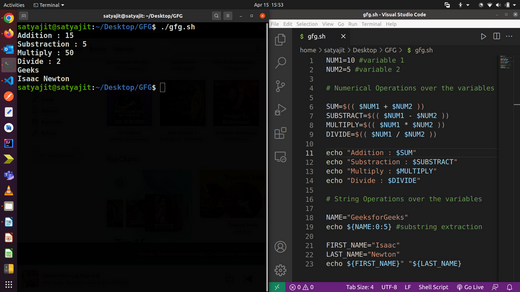## Special Variables

There is some special type of variables present in the bash. These are also called internal variables or preset variables. Below is the list of some of them.

Example Script:

```function Printname(){
echo "First Name : \${1}"
echo "Last Name : \${2}"
echo "Full name is : \$@"
}

Printname Satyajit Ghosh

echo "The name of the user : \$USER"
echo "The hostname of the computer : \$HOSTNAME"
echo "One random number is \$RANDOM"
echo "The process ID of this script : \$\$"```

Output of Special Variables:

```First Name : Satyajit
Last Name : Ghosh
Full name is : Satyajit Ghosh
The name of the user : satyajit
The hostname of the computer : satyajit-ThinkPad
One random number is 3239
The process ID of this script : 4301```

Below is the terminal shell depiction after executing the script –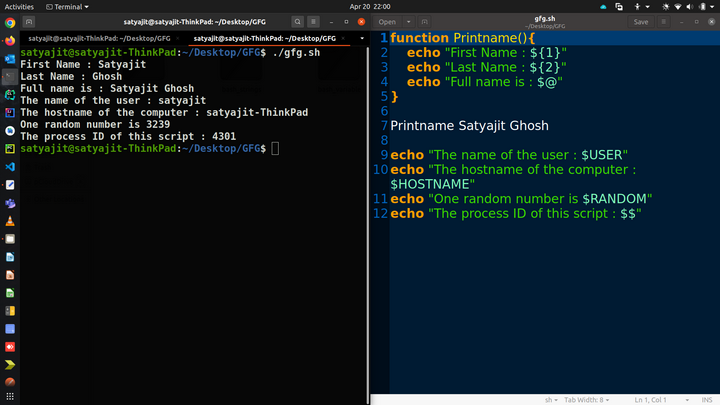## Command-line Arguments

We can supply arguments during the execution of the script and those variables can control the behavior of the script. Suppose we have a script as follows –

Example Script:

echo “The First name is \$1 and Last name is \$2”

Now, if we execute it like this :

`./scriptname.sh arg1 arg2`

We will see the following output –

Output of Command-line Arguments:

The First name is Satyajit and Last name is Ghosh

Below is the terminal shell depiction after executing the script –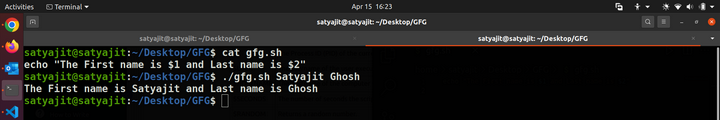## Command Substitution

Command substitution allows us to take the output of a command or program and save it as the value of a variable. Below is the example for the same –

Example Script:

```var=\$( ls /usr | wc -l )
echo Total \$var entries on usr directory```

Output of Command Substitution:

`Total 13 entries on usr directory`

In the above example, we have stored the result of ls /usr | wc -l , in a variable.

Below is the terminal shell depiction after executing the script –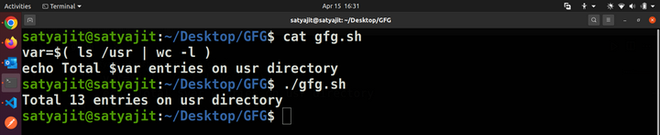## Exporting Variables

Variables are limited to the process they were created in. If we want the variable to be available to another script then we need to export the variable. Below is an example of the same –

Example Script:

First, we will save two files gfg_one.sh and gfg_two.sh, then we will provide the execute permission and have the below codes within them –

gfg_one.sh code:

```NUM1=10

echo "gfg_one : The initial value is \$NUM1"

export NUM1
./gfg_two.sh

echo "gfg_one : The new value is \$NUM1"```

gfg_two.sh code:

```echo "gfg_two : The initial value is \$NUM1"

NUM1=20

echo "gfg_two : The new value is \$NUM1"```

Output of Exporting Variables:

```gfg_one : The initial value is 10
gfg_two : The initial value is 10
gfg_two : The new value is 20
gfg_one : The new value is 10```

So here in this example, we have exported the NUM1 variable from gfg_one.sh and called gfg_two.sh script.

gfg_two.sh receives the NUM1 variable and displayed its value as well ( line 2 of output ).

Now, we can notice one problem in this output in line 4 of the output the value is assumed to be 20 but it is not. Because a copy of the original variable passed to the second script and not the actual one, any change of value in that, will not reflect in the original variable. Below is the terminal shell depiction after executing the script –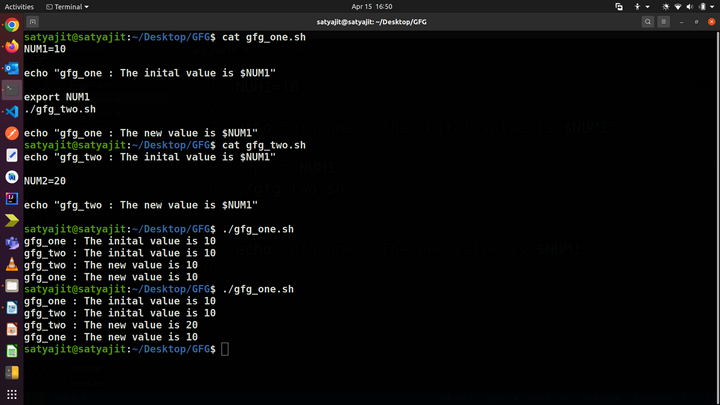My Personal Notes arrow_drop_up